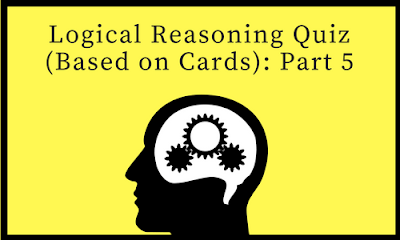Gurpurab Offer - Use Code GURPURAB2023

# Logical Reasoning Quiz (Based on Cards): Part 5#### Directions : Refer to the data below and answer the questions that follow :

Four friends A,B,C and D are playing a card game called FLASH in which 3 cards are dealt to each player. The card value of each card is the number written on it. The face cards Jack , Queen and king have values 11,12,and 13 respectively. The ace card have value one. The players cannot see each others cards. Once a player takes a look at his cards, he can decide whether to continue or quit the game. If the value of each of his cards is less than or equal to 5 , he quit from the game. The remaining players continue the game in which there could be more than one round. There is a showdown (i.e. the cards are shown to the other players )in which the players with maximum card value is declared the winner. A player quitting the game in any round does not have to pay any penalty to the winner. If the showdown is in the first round , the losers have to pay the winner an amount equal to their total card value. But ,if the showdown is in the second round , the losers have to pay the winner an amount twice their total card value and so on.

### Question 1:

In the game A and B quits in the first round while C and D goes into the second round , C decided to have a shutdown , in which D is declared the winner. What is the maximum amount that D could receive from C ?

a) Rs.37
b) Rs.74
c)Rs.36
d) Rs.76

### Question 2:

In a game, where no one quits, the showdown is in the first round and B is declared the winner. What is the minimum amount that B can win ?

a) 15
b) 25
c) 27
d) 26

### Question 3:

In a game, A quits as soon as he takes a look at his cards. The total value of C’s cards is double that of B’s total card value. D is declared the winner. What is the maximum total card value that B can win ?

a) 36
b ) 19
c) 18
d) 15

### Question 4:

In the game, only A quits, The total card value of D is twice that of C and the total card value of C is twice that of B. Which one of the following could be C’s total card value ?

a)`17
b) 19
c) 16
d) 12

### Question 5:

In the game only B quits , as his cards did not fulfill the basic requirement whereas A,C and D proceed to the third round. In the third round C calls for a showdown .D who had a total of 30 points wins the game. None of the players had any face card. Two players had two cards of value 9 each and D won a total of 141.What are the cards with A if C has a card of value one ?

a) 1,1,6
b) 9,9,5
c) 9,9,1
d) 9,9,10

#### 1)

D is declared the winner.
The maximum total value of his cards can be 13+13+13=39, while the maximum total value of C’s cards can 13+12+12 =37. Hence , the maximum amount that D can win from C is 37*2=74.

#### 2)

Since no one quits the game, each person should have at least one card of value more than 5. Thus all the three persons will have only one card of face value 6 each and the remaining cards with each person will be any two from 1,1,1,1,2 and2. therefore the minimum amount that B can win is 26.
Hence ( D)

#### 3)

The maximum total card value will be an even number as it is twice that of B’s total card value .now assuming D has a maximum of 39 point, C can have maximum of 36 points, then B’s maximum total card value will be 18 .
Hence,(C) .

Note : C cannot have 38 (13+13+12)points as D has 39 points (13+13+13).

#### 4)

C’s total card value has to be an even number since it is twice the total card value of B. if C’s total card value is 12,then B’s total card value will be 6.but B cannot be in the game with a total of six points as a person with each card value less than or equal to five has to quit . if C’s total card value is 16’then B’s total card value is 8 i.e.,6+1+1, which is possible and D’s total card value will be 32 which is again possible.
Hence (C)

#### 5)

D has a total of 30 points which does not include any face cards. Therefore , the card with D has to be 10,10,10.D has won a total of rs.141 in the third round which implies that the total card value of A and C together is 141/3=47.A and C each has two 9 card each , which accounts for a total of 36 points . of the remaining 11 points, one point is accounted for by C’s 1 ace card. Therefore, the third card with A has to be 10.
D 10 10 10
A 9 9 10
C 9 9 1
Hence (D).